# Tensorial map

(diff) ← Older revision | Latest revision (diff) | Newer revision → (diff)

## Definition

Suppose$M$ is a differential manifold and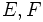$E,F$ are vector bundles over$M$. A$\R$-linear map:$f: \Gamma(E) \to \Gamma(F)$

between the spaces of sections is termed tensorial or pointwise if it satisfies the following equivalent conditions:

1. There exists a linear map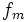$f_m$ from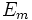$E_m$ to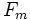$F_m$ for every point$m$, which gives rise to$f$, in the sense that for any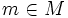$m \in M$ and$s \in \Gamma(E)$: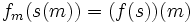$f_m(s(m)) = (f(s))(m)$

1.$f$ is linear with respect to the algebra of all real-valued functions on$M$. In other words, for any real-valued function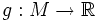$g: M \to \R$ we have: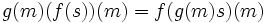$g(m)(f(s))(m) = f(g(m)s)(m)$

1.$f$ is linear with respect to the algebra of all continuous real-valued functions on$M$. In other words, for any continuous real-valued function$g: M \to \R$ we have the above condition.
2.$f$ is linear with respect to the algebra of all smooth ($C^\infty$) functions. In other words, for any smooth real-valued function$g: M \to \R$ we have the above condition